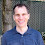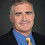## Tuesday, January 31, 2023

### Why does pi come up so often? I don't know either but ...

Here is how history DID unfold:

1) People noticed that the ratio of the circumference to the diameter of ANY circle is always the same, it's a number between 3 and 4, roughly 3.14 or as my Dad would say, exactly 22/7 (see this blog post). On the web (see here) is a claim that Euler first used the symbol pi since it is the first letter of a Greek word for Circumference. I've seen other sites that claim someone less famous and its the first letter of a Greek work for Perimeter

But in any case pi was known (though not called that) a LONG time ago.

2) MUCH LATER people noticed

1/1^2 + 1/2^2 + 1/3^2 + ... = pi^2/6 (Euler showed this in 1735, see here. That site says that it was Basel's problem to determine the sum. Yeah for Basel--- his name lives on even after his problem was solved! This did not happen for Baudet and Vazsonyi. If you don't know what they conjectured--- well, that proves my point. ADDED LATER: commenter Robert pointed out that Basel is NOT a person's name but a cities name. I am delighted to know that!)

1 - 1/3 + 1/5 - 1/7 + 1/9 ... = pi/4 (Leibniz showed this in 1676, see here. A good Quora site on that sum is here.)

(There are other series where pi comes up as well.)

3) People wonder Why did pi, which has to do with CIRCLES, end up in INFINITE SERIES?

What if history unfolded the other way:

1) People noticed

1/1^2 + 1/2^2 + 1/3^2 + ... =a^2/6 (Euler did that in 1735, see here.)

1 - 1/3 + 1/5 - 1/7 + 1/9 ... = b/4 (Leibniz showed this, see here. A good Quora site on that sum is here.)

and they noticce that a=b and is between 3 and 4, closer to 3. They decide to call it pi for no good reason.

(There are other series where pi comes up as well.)

2) MUCH LATER people noticed that this same funny constant pi was the ratio of circumference to diameter in any circle.

3) People wonder Why did pi, which has to do with INFINITE SERIES, end up in CIRCLES?

The following comic captures the dichotomy: here

1.One might argue that 2 pi is more fundamental.

1.Indeed, it has been argued in The Tau Manifesto:

https://tauday.com/tau-manifesto

2.My favorite pi claim is that of the Tau Manifesto, namely that pi is the wrong circle constant. Like all good jokes, it has a lot of truth to it. The basic point is that pi is NOT the "circle constant", it's the "half circle constant". That is, pi radians only gets you half way around the circle, it takes TAU radians to get you back to where you started.

So Euler's formula, e^ipi = -1 is bletcherous because the real beauty here is that TAU gets you all the way around, so e^iTAU = 1. There are lots of formulas where an egregious factor of 2 shows up, and those are pretty much always because we're using pi instead of the correct circle constant TAU. (Somewhat seriously, when I red the Tau Manifetso, I got a way better understanding of trig than I had doing (non-discrete) math through the required calculus courses and E&M physics.)

To take this one step further, consider the Voyager space probes. The folks at NASA put a whole bunch of writing on it's outer hull so that if any aliens found it, they'd realize how smart we were. Including pi to a ridiculous number of decimal places. But what if said aliens had not made the mistake of using a circle constant half the sensible value and did their calculus and the like using TAU. They'd think we were idiots.

Finally, to get back to the posted question, there are YouTube videos that explain how and why pi appears in infinite series. Here's one:

1.thanks for videos!

The aliens would think we were idiots. Would they then help us or hurt us or ignore us or what? Not expecting an answer, just thought I would bring it up.

3.In your alternate history, someone looked at the sequence 1/1^2 + 1/2^2 + 1/3^2 + ... = u =1.64993... and someone else looked at the not-so-obvious sequence 1 - 1/3 + 1/5 - 1/7 + 1/9 = v = 0.785398... and then someone somehow realized, u = 8/3 v^2, which must be important. I'm just not seeing history develop that way.

1.Excellent point -- it shows, why people should be interested about algebraic independence of constants https://en.wikipedia.org/wiki/Algebraic_independence
Somehow, it sounded to arbitrary to me, before seeing this way of looking at $\pi$ and $\pi^2$.

4.Some alternative histories are less believable than others. I would agree that mine is on the less-believable side of the spectrum. There are more believable examples of `math could have been done in a diff order' I would blog on that, though I probably already have.

5.In a hand-wavy way if we think of a circle as the lim-sup of regular polyhedrons with number of edges doubling in each iteration the circumference (or area) of the circle could be written out as summation of difference between two adjacent polyhedrons in the sequence....

1.Some of the earliest notions of limits involve approximations to pi shown by Archimedes (see e.g., https://arxiv.org/ftp/arxiv/papers/2008/2008.07995.pdf which also has earlier references to series involving pi) and it seems that doing this motivated him to develop very calculus-like ideas (see https://www.pbs.org/wgbh/nova/archimedes/ on their rediscovery). There was no printing press to disseminate ideas quickly but what if that had been followed up on by others at the time?

2.6.